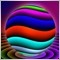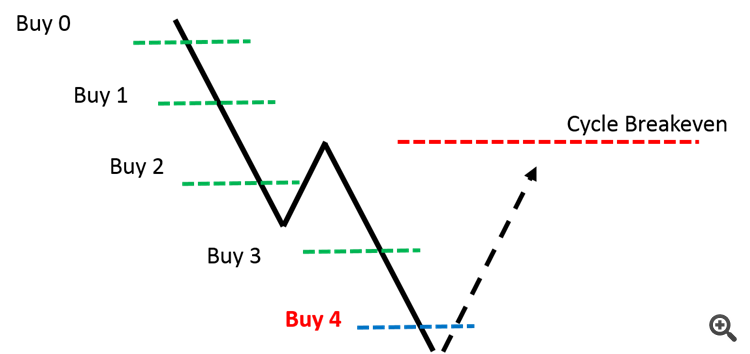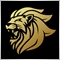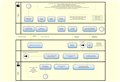# Design map for newbie in mql41802

Hi,

I am trying to code a user defined function in which I would like to calculate the required lot size of the last martingale order in a cycle to achieve breakeven on certain price , upon reversal.

For example, Buy orders 0, 1,2, and 3 were already opened while the price going down. Now, I want to open Buy 4 order. I need to calculate its size to achieve BE on the red price

I do not need a code but a thinking map of how I can do it. Then, I will try myself and post here to see if it right or wrong.20228

Hatem Abou Ouf: I need to calculate its size to achieve BE on the red price
BE (price) is lots weighted average price (Ei loti*pricei)/(Ei loti) Do the algebra.Moderator
12729

Hatem Abou Ouf:

Hi,

a thinking map

Well the closest to what i can think of is a flow chart here: http://draw.iodraw.io - free flowchart maker and diagrams online
• draw.io
draw.io (formerly Diagramly) is free online diagram software. You can use it as a flowchart maker, network diagram software, to create UML online, as an ER diagram tool, to design database schema...1802

whroeder1:
BE (price) is lots weighted average price (Ei loti*pricei)/(Ei loti) Do the algebra.

Thank you whroeder1

This is my first trial. If correct, I plan to make my function better by :

1- To make the same function work for both buy and sell cycle orders ( by passing the cycle order type), I think

2- To check better for errors from OrderSelect

This is my first serious week to start mql4 coding just because I like coding!. My plan is to deal with one coding concept per week. ( 15-20 min/daily).

```input int BuyMagic = 1111;
//+------------------------------------------------------------------+
//| Expert initialization function                                   |
//+------------------------------------------------------------------+
int OnInit()
{
//---

//---
return(INIT_SUCCEEDED);
}
//+------------------------------------------------------------------+
//| Expert deinitialization function                                 |
//+------------------------------------------------------------------+
void OnDeinit(const int reason)
{
//---

}
//+------------------------------------------------------------------+
//| Expert tick function                                             |
//+------------------------------------------------------------------+
void OnTick()
{
double BE_Price = 1.2345;

}
//+------------------------------------------------------------------+

//---------------------------------------------------------------------------------+
// Calculate the Required lot                                                      |
//---------------------------------------------------------------------------------+
{
//----
double Lots   = 0.0;                                      // to calculate the sum of all lots except the current
double OrderOPrice = 0.0;                                 // to calculate the OP of all open orders
double LotsPrice = 0.0;                                   //
double OP_CurrentBuy = MarketInfo(Symbol(), MODE_BID);    // Open price of the current buy order
double Average = BE_Price;                         // pass it to the function
double Required_Lot;

for (int i = OrdersTotal() - 1; i >= 0; i--)
{
if (OrderSymbol() == Symbol() && OrderType() == OP_BUY && OrderMagicNumber() == BuyMagic)
{
Lots = Lots + OrderLots();
LotsPrice = LotsPrice + OrderLots()*OrderOpenPrice();

Print("Lots : ", OrderLots(), "   OrderOpenPrice : ", OrderOpenPrice());
}
}
Required_Lot = NormalizeDouble (LotsPrice - (Average *Lots)/ (Average - OP_CurrentBuy),Digits) ; // Old Old Old Algebra rusty mind :)

//----
return(Required_Lot);
}```1802

Oh, I forget to account for the cost of transactions ( Commission and swap). I will try to include that. If you have a clue for me, please do not not hesitate to mention.1802

Marco vd Heijden:

Well the closest to what i can think of is a flow chart here: http://draw.io

Very interesting tool. Thanks for sharing20228

Hatem Abou Ouf: This is my first trial. If correct, I plan to make my function better by :
`Required_Lot = NormalizeDouble (LotsPrice - (Average *Lots)/ (Average - OP_CurrentBuy),Digits);`

Close but not quite.

1. Misplaced parenthesis
```BE = (Σ(lot*price) + newLot newPrice) / (Σ(lot) + newLot)
BE (Σ(lot) + newLot)= (Σ(lot*price) + newLot newPrice)
BE (Σ(lot) + newLot) - newLot newPrice = Σ(lot*price)
BE newLot - newLot newPrice = Σ(lot*price) - BE Σ(lot)
newLot (BE - newPrice) = Σ(lot*price) - BE Σ(lot)
newLot = {Σ(lot*price) - BE Σ(lot)} / (BE - newPrice)

Required_Lot = {LotsPrice  - Average lots} / (Average - OP_CurrentBuy)```

2. Do NOT use NormalizeDouble, EVER. For ANY Reason. It's a kludge, don't use it. It's use is usually wrong, as it is in this case.